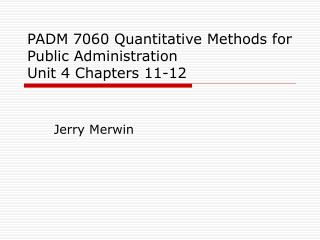# PADM 7060 Quantitative Methods for Public Administration Unit 4 Chapters 11-12 - PowerPoint PPT PresentationDownload PresentationDownload Presentation## PADM 7060 Quantitative Methods for Public Administration Unit 4 Chapters 11-12

- - - - - - - - - - - - - - - - - - - - - - - - - - - E N D - - - - - - - - - - - - - - - - - - - - - - - - - - -
##### Presentation Transcript

1. PADM 7060 Quantitative Methods for Public AdministrationUnit 4 Chapters 11-12 Jerry Merwin

2. Meier, Brudney & Bohte Part IV: Inferential Statistics • Unit 4 • Chapter 11: Introduction to Inference • Chapter 12: Hypothesis Testing • Unit 5 • Chapter 13: Estimating Population Proportions • Chapter 14: Testing the Difference Between Two Groups

3. Meier, Brudney & Bohte: Chapter 11 Introduction to Inference • Explain the difference between descriptive statistics and inferential statistics.

4. Meier, Brudney & Bohte: Chapter 11 Introduction to Inference (Page 2) • What are the basic concepts and definitions associated with inferential statistics? • Population • Parameters • Sample • Random selection • Statistic

5. Meier, Brudney & Bohte : Chapter 11 Introduction to Inference (Page 3) • How important is random selection to the concept of inference? • What are the difference in parameters and statistics? • Symbols (See table 11.1) • Calculations • Mean? • Standard deviation?

6. Meier, Brudney & Bohte: Chapter 11 Introduction to Inference (Page 4) • How do we estimate the population mean? (177) • What do we mean by sampling error? • How can we reduce sampling error? • Ideal sample size is n  30.

7. Meier, Brudney & Bohte: Chapter 11 Introduction to Inference (Page 5) • How do we estimate the population standard deviation? (see pages 178-179) • Why do we calculate s with the denominator n-1? • How can we reduce sampling error?

8. Meier, Brudney & Bohte: Chapter 11 Introduction to Inference (Page 6) • What is the standard error of the mean? (pages 179-180) • How can we estimate the standard error of the mean without taking many samples? • See the formula on page 181 • Also, note the explanation about using the estimated standard deviation in the computations.

9. Meier, Brudney & Bohte: Chapter 11 Introduction to Inference (Page 7) • Let’s look at the example on page 180 regarding the Yukon police: • The calculations for standard error of the mean are on page 181 • Next, we will talk about how we can use this information.

10. Meier, Brudney & Bohte: Chapter 11 Introduction to Inference(Page 8) • What is the “Student’s t distribution” (a.k.a. the t distribution) • Characteristics: • With n > = 30 normal distribution works • Resembles normal distribution but flatter • Differs for each sample size • Need to know the degrees of freedom (df)

11. Meier, Brudney & Bohte: Chapter 11 Introduction to Inference(Page 9) • What is a confidence limit? • How is it calculated? • See formula • Using our example from Yukon: • First look up the t values in table 3 on 446 (see book about why we use .025 and d.f. = 4) • With the formula, we get • 15.6 + (1.7 x 2.78) = 20.3 for the upper limit • 15.6 – (1.7 x 2.78) = 10.9 for the lower limit

12. Meier, Brudney & Bohte: Chapter 11 Introduction to Inference(Page 10) • With this information, what does it tell us about the confidence limit? • 15.6 + (1.7 x 2.78) = 20.3 for the upper limit • 15.6 – (1.7 x 2.78) = 10.9 for the lower limit • We are 95% confident that…

13. Meier, Brudney & Bohte: Chapter 11 Introduction to Inference(Page 11) • Problems 11.2, 11.6 11.8

14. Meier, Brudney & Bohte : Chapter 12 Hypothesis Testing • How is hypothesis testing related to theory and research?(Diagram courtesy of Dr. Nolan J. Argyle, from the text listed below) David Nachmias & Chava Nachmias, Research Methods in the Social Sciences, 2nd ed. New York: St. Martins Press, 1981, p. 23.

15. Meier, Brudney & Bohte: Chapter 12 Hypothesis Testing (Page 2) • What is a hypothesis? • How is a hypothesis important to a manager? • Explain the null hypothesis?

16. Meier, Brudney & Bohte: Chapter 12 Hypothesis Testing(Page 3) • What are the steps in hypothesis testing? • Formulate the hypothesis • Collect the relevant data • Evaluate the hypothesis in light of the data • Accept or reject the hypothesis • Revise your decisions in light of the new information

17. Meier, Brudney & Bohte: Chapter 12 Hypothesis Testing(Page 4) • What is the primary type of hypothesis testing we will do in managerial situations? • Hypothesis testing with samples! • Example from Prudeville

18. Meier, Brudney & Bohte: Chapter 12 Hypothesis Testing(Page 5) • Who can explain the concept of one-tailed and two-tailed tests? • When do we use the one-tailed test? • Figure 12.1

19. Meier, Brudney & Bohte: Chapter 12 Hypothesis Testing(Page 6) • What are the two types of errors we can make testing a null hypothesis? • Type 1 • Type 2 • How can we decrease type 1 errors?

20. Meier, Brudney & Bohte: Chapter 12 Hypothesis Testing(Page 7) • How can we determine sample size? • Consider: • The amount of error that can be tolerated • The confidence one wants to have in the error estimate • The standard deviation of the population

21. Meier, Brudney & Bohte: Chapter 12 Hypothesis Testing(Page 8) • Problems 12.2, 12.6